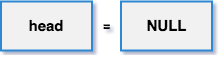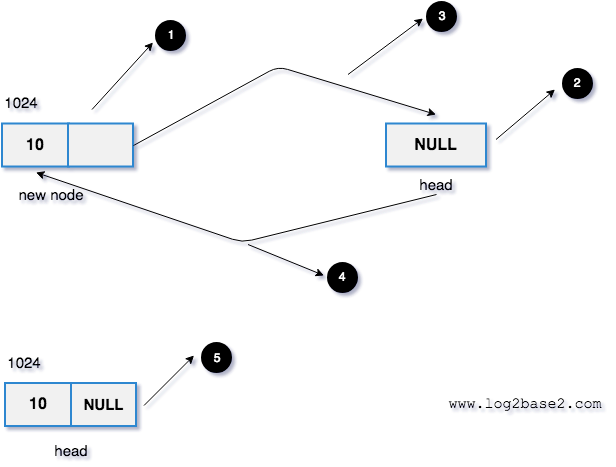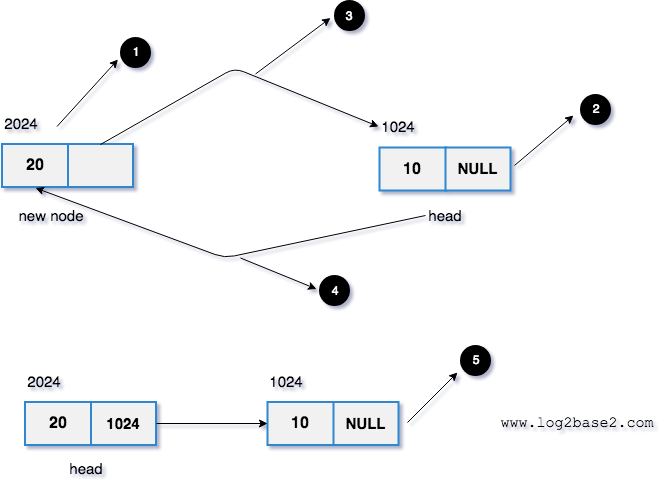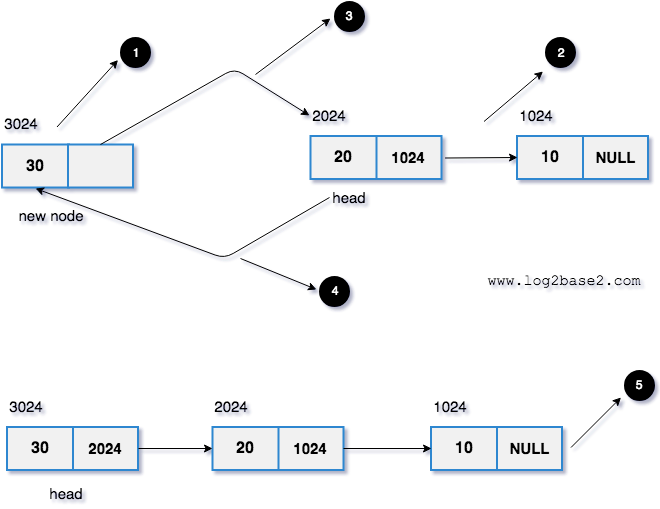# Inserting a node at the beginning of a linked list

The new node will be added at the beginning of a linked list.

#### Example

Assume that the linked list has elements: 20 30 40 NULL

If we insert 100, it will be added at the beginning of a linked list.

After insertion, the new linked list will be

100 20 30 40 NULL

## Algorithm

1. Declare a head pointer and make it as NULL.

2. Create a new node with the given data.

3. Make the new node points to the head node.

4. Finally, make the new node as the head node.

## 1. Declare a head pointer and make it as NULL

```struct node
{
int data;
struct node *next;
};

```

## 2.Create a new node with the given data.

```void addFirst(struct node **head, int val)
{
//create a new node

struct node *newNode = malloc(sizeof(struct node));
newNode->data = val;

}
```

## 3 .Make the newnode points to the head node

```void addFirst(struct node **head, int val)
{
//create a new node
struct node *newNode = malloc(sizeof(struct node));
newNode->data = val;

}
```

## 4. Make the new node as the head node.

```void addFirst(struct node **head, int val)
{
//create a new node
struct node *newNode = malloc(sizeof(struct node));
newNode->data = val;

}
```

## Visual Representation#### Let's insert data 10.1. A newly allocated node with data as 10.

3. New node -> next points to the head which is NULL. So newnode->next = NULL.

4. Make the head points to the new node. Now, the head will hold the address of the new node which is 1024.

5. Finally, the new linked list.

#### Let's insert data 20.1. A newly allocated node with data as 20.

2. Head points to the memory address 1024 (It has only one node. 10->NULL).

3. New node -> next points to the head which is 1024. So newnode->next = 1024 (10->NULL) will be added back to the new node.

4. Make the head points to the new node. Now, the head will hold the address of the new node which is 2024.

5. Finally, the new linked list.

#### Let's insert data 30.1. A newly allocated node with data as 30.

2. Head points to the memory address 2024 (It has two nodes. 20->10->NULL).

3. New node -> next points to the head which is 2024. So newnode->next = 2024 (20->10->NULL) will be added back to the new node.

4. Make the head points to the new node. Now, the head will hold the address of the new node which is 3024.

5. Finally, the new linked list.

## Program to insert a node at the beginning of linked list

Example

```#include<stdio.h>
#include<stdlib.h>

struct node
{
int data;
struct node *next;
};

{
//create a new node
struct node *newNode = malloc(sizeof(struct node));
newNode->data = val;

}

{

//iterate the entire linked list and print the data
while(temp != NULL)
{
printf("%d->", temp->data);
temp = temp->next;
}
printf("NULL\n");
}

int main()
{